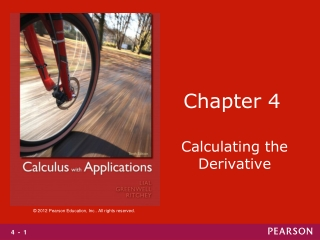DownloadDownload PresentationChapter 4

# Chapter 4

Télécharger la présentation## Chapter 4

- - - - - - - - - - - - - - - - - - - - - - - - - - - E N D - - - - - - - - - - - - - - - - - - - - - - - - - - -
##### Presentation Transcript

1. Chapter 4 Calculating the Derivative

2. Section 4.1 Techniques for Finding Derivatives

4. Your Turn 2 Solution: Rewrite this as,

5. Your Turn 3 Solution: Rewrite h(t) as

6. Figure 4

7. Figure 5

8. Figure 6

9. Section 4.2 Derivatives of Products and Quotients

10. Your Turn 1 Solution : Simplify by multiplying and combining terms.

12. Section 4.3 The Chain Rule

13. Figure 9

15. Your Turn 6 Solution: Now use the product rule and the chain rule.

16. Section 4.4 Derivatives of Exponential Functions